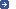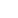– the intergovernmental organization through which Member States act together
on matters related to measurement science and measurement standards.
 Search facility:| Site map | News | Contact us | [ FR ]Chemistry and Biology: SI base unit (mole)Definition agreed by the 26th CGPM (November 2018), implemented 20 May 2019:

The mole, symbol mol, is the SI unit of amount of substance. One mole contains exactly 6.022 140 76 x 1023 elementary entities. This number is the fixed numerical value of the Avogadro constant, NA, when expressed in the unit mol–1 and is called the Avogadro number.

The amount of substance, symbol n, of a system is a measure of the number of specified elementary entities. An elementary entity may be an atom, a molecule, an ion, an electron, any other particle or specified group of particles.This definition implies the exact relation NA = 6.022 140 76 x 1023 mol–1. Inverting this relation gives an exact expression for the mole in terms of the defining constant NA:The effect of this definition is that the mole is the amount of substance of a system that contains 6.022 140 76 x 1023 specified elementary entities.Decisions relating to the base units of the SI SI Brochure, from Appendix 1Amount of substance:14th CGPM, 1971 definition of the mole, symbol mol, as a seventh base unit, and roles for its use21st CGPM, 1999 adopts the special name katal, kat23rd CGPM, 2007 on the possible redefinition of certain base units of the International System of Units (SI)24th CGPM, 2011 on the possible future revision of the International System of Units, the SI25th CGPM, 2014 on the future revision of the International System of Units, the SI26th CGPM, 2018 revision of the International System of Units, the SI

Following the discovery of the fundamental laws of chemistry, units called, for example, "gram-atom" and "gram molecule", were used to specify amounts of chemical elements or compounds. These units had a direct connection with "atomic weights" and "molecular weights", which are in fact relative atomic and molecular masses. The first compilations of "Atomic weights" were originally linked to the atomic weight of oxygen, which was, by general agreement, taken as being 16. Whereas physicists separated the isotopes in a mass spectrometer and attributed the value 16 to one of the isotopes of oxygen, chemists attributed the same value to the (slightly variable) mixture of isotopes 16, 17 and 18, which for them constituted the naturally occurring element oxygen. An agreement between the International Union of Pure and Applied Physics (IUPAP) and the International Union of Pure and Applied Chemistry (IUPAC) brought this duality to an end in 1959-1960. Physicists and chemists had agreed to assign the value 12, exactly, to the so-called atomic weight, correctly referred to as the relative atomic mass Ar, of the isotope of carbon with mass number 12 (carbon 12, 12C). The unified scale thus obtained gives the relative atomic and molecular masses, also known as the atomic and molecular weights, respectively. This agreement is unaffected by the redefinition of the mole.

The quantity used by chemists to specify the amount of chemical elements or compounds is called "amount of substance". Amount of substance, symbol n, is defined to be proportional to the number of specified elementary entities N in a sample, the proportionality constant being a universal constant which is the same for all entities. The proportionality constant is the reciprocal of the Avogadro constant NA, so that n = N/NA. The unit of amount of substance is called the mole, symbol mol. Following proposals by the IUPAP, IUPAC and ISO, the CIPM developed a definition of the mole in 1967 and confirmed it in 1969, by specifying that the molar mass of carbon 12 should be exactly 0.012 kg/mol. This allowed the amount of substance nS(X) of any pure sample S of entity X to be determined directly from the mass of the sample mS and the molar mass M(X) of entity X, the molar mass being determined from its relative atomic mass Ar (atomic or molecular weight) without the need for a precise knowledge of the Avogadro constant, by using the relations

nS(X) = mS/M(X), and M(X) = Ar(X) g/mol

Thus, this definition of the mole was dependent on the artefact definition of the kilogram.

The numerical value of the Avogadro constant defined in this way was equal to the number of atoms in 12 grams of carbon 12. However, because of recent technological advances, this number is now known with such precision that a simpler and more universal definition of the mole has become possible, namely, by specifying exactly the number of entities in one mole of any substance, thus fixing the numerical value of the Avogadro constant. This has the effect that the new definition of the mole and the value of the Avogadro constant are no longer dependent on the definition of the kilogram. The distinction between the fundamentally different quantities 'amount of substance' and 'mass' is thereby emphasized. The present definition of the mole based on a fixed numerical value for the Avogadro constant,NA, was adopted in Resolution 1 of the 26th CGPM (2018).See more: History of the SIBIPM - Pavillon de Breteuil F-92312 Sèvres Cedex FRANCE DisclaimerPrivacy Policy Copyright © BIPM all rights reserved